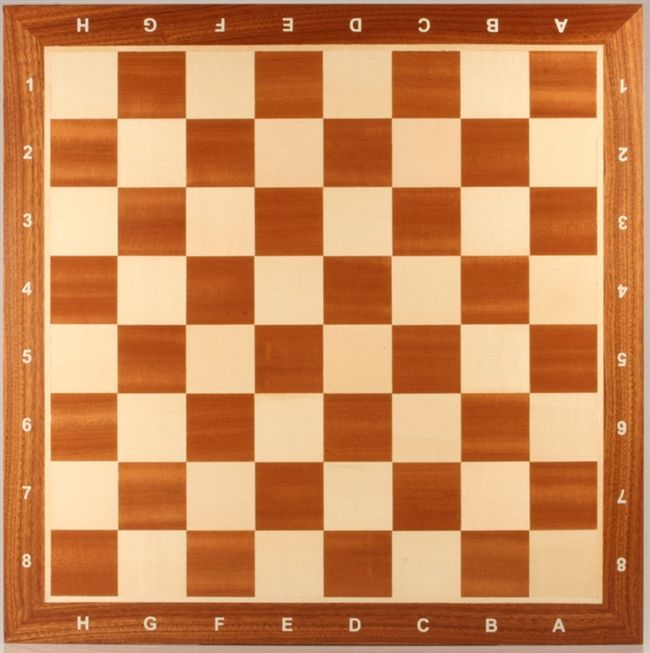# Walking Across a Chess Board

Probability Level 5One day, Bobby decided that it might be a good idea to take a nice stroll across the giant chess board that he built in his back yard. He starts at the bottom left corner, walks in a straight line, and crosses exactly 12 squares. Technically, there are infinitely many possible directions that Bobby could have walked in.

However, if we restrict Bobby's path to lines with slopes of the form $\frac{a}{b}$, where $a are positive coprime integers, then the number of solutions is finite. Under this constraint, how many possible solutions are there?

×